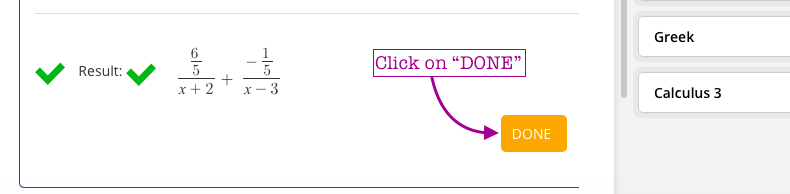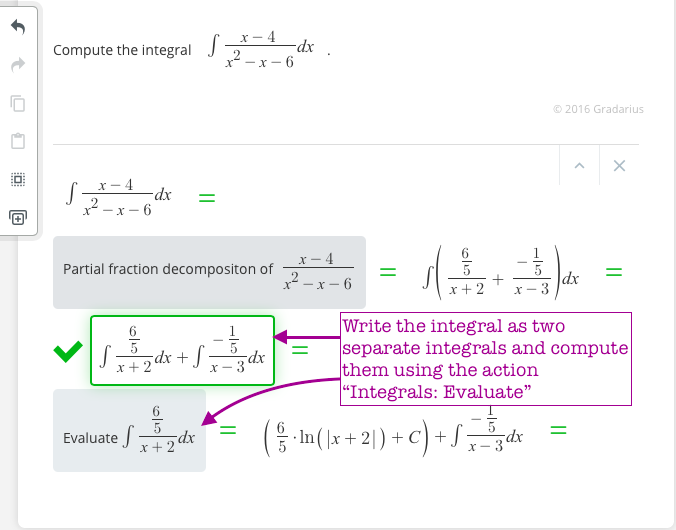The method of partial fractions is used to integrate rational functions with the degree of the polynomial in the numerator is less than degree of the polynomial in the denominator.  Note that if the degree of the polynomial in the numerator is greater or equal to the degree of the polynomial in the denominator then we first have to do a polynomial long division

Let's integrate the following function using this method:We leave it to the reader to complete the rest of the integral.